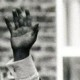# The proof that frightened Pythagoras

I have been meaning to do this for a while …

Pythagoras was said to be shocked by the proof that$\sqrt 2$ is an irrational number (i.e., that it cannot be represented as a ratio of two whole numbers). The proof (by contradiction) is a simple one.

If$\sqrt 2$ is rational then$\sqrt 2 = \frac{a}{b}$ and$2 = \frac{a^2}{b^2}$ where$a$ and$b$ are the smallest nominator and denominator possible, i.e, in the lowest terms.

Hence$a^2 = 2b^2$ and so$a$ must be even (as two odd numbers multiplied always give an odd number – let$l = 2x$ and$m = 2y$ be even numbers then$(l + 1)(m + 1) = lm + l + m + 1$). Hence$a = 2k$.

But we also have$4k^2 = a^2$ and thus$4k^2 = 2b^2$ and$b^2 = 2k^2$ and so$b$ is even.

So we have$a$ and$b$ sharing a common factor of 2, so they cannot be the nominator and denominator of the fraction in the lowest terms.

But if$a$ and$b$ are both even then they share a common factor of 2 and$\frac{a}{b} = \frac{2k}{2p} = \frac{k}{p}$: implying that$a = k$ and$a = 2k$, an obvious contradiction: hence$\sqrt 2$ cannot be a rational.

Update: I have made the final step of this shorter and clearer.

Further update: I have been told (see comments below) I would have been better sticking with a clearer version of the original ending ie., we state that$\frac{a}{b}$ are the lowest terms. Then, plainly as they have a factor in common (2) they cannot be the lowest terms and so we have a contradiction. Would be great if someone could explain why we cannot use the$a = 2k = k$ contradiction.

And another update: Should have stuck with the original explanation – which I have now restored in a hopefully clearer way. The comments below are really interesting and from serious mathematicians, so please have a look!

## 9 thoughts on “The proof that frightened Pythagoras”

1.castover says:

In your last step, you deduce that$a=k$ from$\frac{a}{b}=\frac{k}{p}$, which isn’t necessarily correct. The only deduction that holds is that$ap=bk$.

The part of this proof that usually lends the contradiction is from the fact that$\frac{a}{b}$ would have a 2 in common. In particular, an earlier step in the proof is to generally assume that$\sqrt{2}=\frac{a}{b}$ where$a$ and$b$ are in lowest terms (and hence have no common factors).

This was one of my favorite proofs as an undergraduate: Thanks for taking the time to share it with others. 🙂

1.Adrian McMenamin says:

Thanks. Not a maths graduate, so happy to accept I have got it wrong, but would like to know more 🙂 I did, essentially, have a version of the fact they were in lowest terms before and then changed it to current version – why doesn’t that necessarily hold?

1.castover says:

Sorry for not responding sooner; I haven’t been around much today.

The short answer as to why that doesn’t necessarily hold can be shown by an example:$\frac{1}{2}=\frac{3}{6}$ though clearly$1\neq 3$. As mentioned above, however,$6(1)=3(2)$.

The longer answer is from abstract algebra: Given a commutative ring with identity$R$, its field of fractions$D^{-1}R$ is constructed by “attaching” to$R$ the set$D^{-1}$ of multiplicative inverses (namely, reciprocals) of nonzero elements of$R$. This definition is well-defined precisely when the construction is made as an equivalence class in which elements$\frac{a}{b}$ and$\frac{c}{d}$ are considered equal whenever$ad=bc$.

Given this construction, the answer for your specific proof lies in the fact that$\mathbb{Q}$ is the field of fractions of$\mathbb{Z}$, and so uniqueness of “fractions” (elements of$\mathbb{Q}$) exists only up to the equivalence mentioned above.

If you ever want to talk about this – or anything mathematical, really – further, don’t hesitate to let me know! 🙂

2.Adrian McMenamin says:

Err, yes. I’ve been a bit silly really. Thanks

3.castover says:

Also, in my explanation of$D^{-1}R$, I also apparently neglected to mention that if$R$ has zero divisors, they also can’t be given reciprocals. To be precise, the definition I gave sort of implies that$R$ is assumed to have been an integral domain, though the more general construction for$D^{-1}R$ is done similarly. For details, check here:

http://en.wikipedia.org/wiki/Field_of_fractions

2.Lubos Motl says:

One can also quickly complete the proof by saying that a^2 = 2b^2 is impossible because in the factorization of the left hand side to primes, each prime is exponentiated to an even exponent, while the decomposition of the right hand side to a product of primes has an odd power of 2 (because of the extra power of two), so they can’t be equal. One needs to use that the factorization exists for each positive integer and is unique.

1.Adrian McMenamin says:

Yes, thanks.

3.prubin73 says:

“Would be great if someone could explain why we cannot use the a = 2k = k contradiction.” If a/b = c/d and a/b = e/f, it does not imply that c = e and d = f UNLESS both c/d and e/f are in lowest terms. So going from a/b = k/p to a = k without the lowest terms bit is an unjustified leap.

1.Adrian McMenamin says:

I suppose that is pretty obvious really. Not sure why i let it pass me by. Thanks